#### You may also like### Chocolate

There are three tables in a room with blocks of chocolate on each. Where would be the best place for each child in the class to sit if they came in one at a time?### Four Triangles Puzzle

Cut four triangles from a square as shown in the picture. How many different shapes can you make by fitting the four triangles back together?### Cut it Out

Can you dissect an equilateral triangle into 6 smaller ones? What number of smaller equilateral triangles is it NOT possible to dissect a larger equilateral triangle into?

# Fractional Triangles

##### Age 7 to 11Challenge Level

Chris and Jenny sent us some colourful diagrams to show their answers: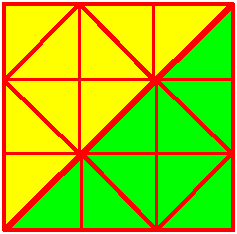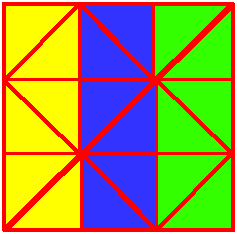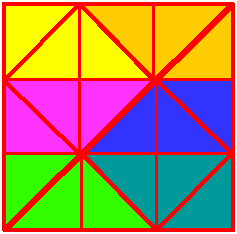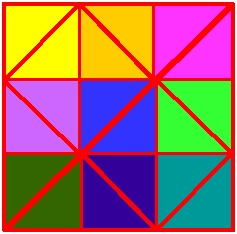Julia drew on the line she'd draw to get quarters. Can you see that there are now four identical triangles? (Concentrate on the thickest lines.)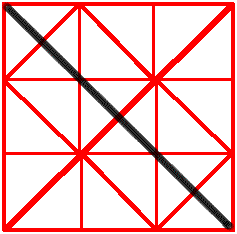However, Anna wrote to say:
The least amount of line needed is one line dividing the central two triangles of the square in half.

I knew that the diagram had 18 triangles which is a number not divisible by 4. So I divided two triangles in half to have a total of 20 triangles which is a number divisible by 4.  At first I drew the smaller triangles at the side of the square but this did not work.  Then I divided all of the triangles in half using 9 lines giving me 36 triangles.  I successfully divided the diagram into equally sized quarters of the same shape, but this did not give me the smallest amount of line.  Once more I split two triangles in half but this time the halved triangle was in the middle.

There are lots of ways to divide the shape up into two halves. Sunita and Raj sent us two that they thought were nice: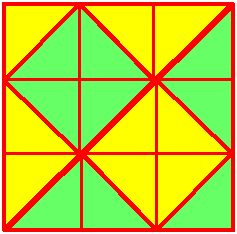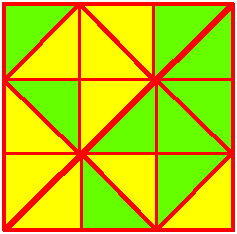The Maths Challenge Group at St Aidan's VC School also sent us some different ways of dividing the shape into two halves.  Here are the images they sent: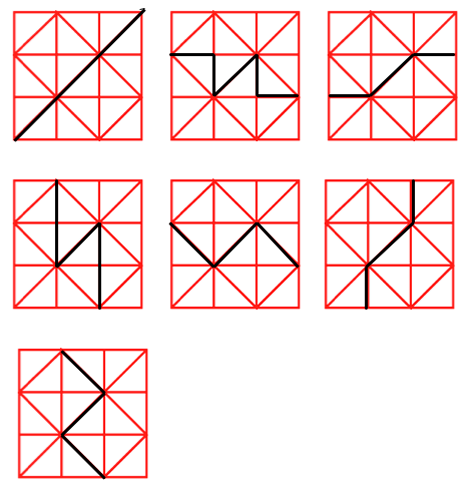They told us:

We all compared our answers and came up with these as our final results. We looked very carefully at  5 and 7, 3 and 6 in order to be sure that they were different answers not merely a rotation. We are happy with our results after our discussion.

Thank you for sharing that with us and well done everyone.